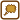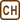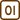## Laboratory Problem

### Task number: 1291

In the morning, when the room temperature was at 20 °C, a careful laboratory worker filled a graduated cylinder with exactly 100 ml of water. During summer, the temperature in the laboratory increased to 30 °C. If we neglected the evaporation of the water and considered only the thermal expansion of water and glass, would the water level be below the line or above the line indicating the volume of 100 ml?

• #### Hint

The volume of the water will increase with the increasing temperature, but the graduated cylinder will also expand. Our task is to find out which volume increases more, whether the water volume or the inner volume of the graduated cylinder.

• #### Numerical values

 VC = 100 ml the volume of water in the graduated cylinder t1 = 20 °C the initial temperature t2 = 30 °C the final temperature From The Handbook of Chemistry and Physics βw = 0.2·10−3K−1 the coefficient of volumetric thermal expansion of water αg = 8·10−6K−1 tthe coefficient of linear thermal expansion of glass
• #### Analysis

At first glance, it might seem that the task has a completely clear solution. When the temperature increases, the water volume also increases, so the water level will be above the line. However, on closer examination we will realize that not only the water but also the glass expands with the increasing temperature, so when the temperature increases, the inner volume of the graduated cylinder also increases. If the expansion of glass outweighed the expansion of water, the water level would be below the line. Our task is therefore to compare these two contradictory effects.

• #### Solution

First we will determine the volume of water at the higher temperature. For the volumetric expansion of water the following formula is true

$V_w = V_C (1 + \beta_W\Delta t).$

Furthermore, we will determine the internal volume of the graduated cylinder at the higher temperature. In the Handbook of Chemistry and Physics, however, there is only the linear expansion coefficient listed. We will therefore have to use the formula for the volume of the cylinder V = πr2h, where r is the radius of the cylinder and h is its height. We will mark the dimensions of the cylinder at the initial temperature with the same letters and differentiate them with index 0.

We will now substitute the relations expressing the change in the dimensions with the increasing temperature into this formula:

$r = r_0 (1 + \alpha_g\Delta t)$ $h = h_0 (1 + \alpha_g\Delta t)$ $V_g=\pi r^2 h=\pi r_0^2(1+\alpha_g\Delta t)^2h_0(1+\alpha_g\Delta t)$ $V_g=\pi r_0^2h_0(1+\alpha_g\Delta t)^3$ $V_g=V_C(1+\alpha_g\Delta t)^3$

We will multiply out the parentheses and realize that the members with α with higher exponent will be in respect to α and Δt too small, and therefore can be neglected:

$V_g=V_C(1 + 3\alpha_g\Delta t+3\alpha_g^2\Delta^2 t+3\alpha_g^3\Delta t^3)$

The last equation shows that with sufficient accuracy the following relationship is true between the thermal linear expansion coefficient αg and the thermal volumetric expansion coefficient βg:

$\beta_g\dot{=}3\alpha _g.$

Our task is therefore to compare the volumes expressed by the relations

$V_w = V_C (1 + \beta_w\Delta t),$ $V_g = V_C (1 + 3\alpha_g\Delta t).$

It is true that βW > 3 αg, We can therefore say that the volume of water increases more than the inner volume of the graduated cylinder. The water level will therefore be above the line.

Let us substitute the numerical values:

$V_w = V_C (1 + \beta_w\Delta t)=100\cdot[1+2\cdot{10^{-4}}\cdot(30-20)]\,\mathrm{ml}$ $V_w = 100.2\,\mathrm{ml}$ $V_g = V_C (1 + 3\alpha_g\Delta t)=100\cdot[1+3\cdot{8}\cdot{10^{-6}}\cdot(30-20)]\,\mathrm{ml}$ $V_g=100.024\,\mathrm{ml}$

Now we can see that the internal volume of the graduated cylinder increases by a smaller value than the internal volume of water and the water will therefore be above the line indicating the volume of 100 ml.

• #### Answer

After the temperature increase the water level will be above the line.
• #### Note – the relative thermal expansion

The thermal expansion of the glass is very small (especially for certain types of glass such as silica glass), and can therefore be neglected in most situations.